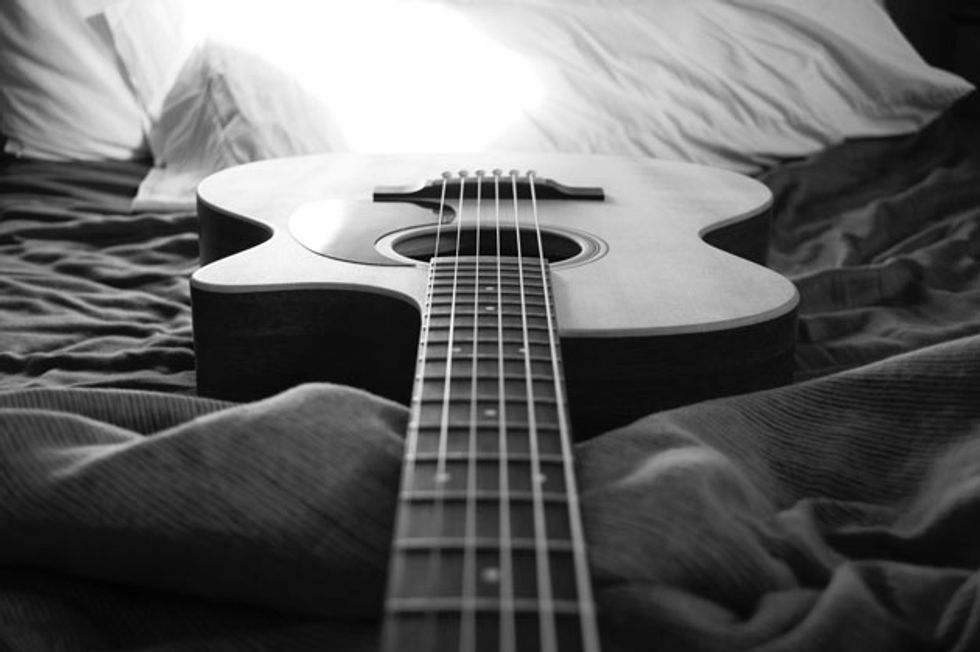Chops: Beginner
Theory: Beginner
Lesson Overview:
• Develop a deeper understanding of chord theory.
• Learn how to systematically move through each key.
• Create a visual map of the entire fretboard.

The main focus of this column is improvisation. We’ll be looking at things from a jazz improv viewpoint, but you can apply these concepts to any style of music. If you want to know how to sound consistently good when writing melodies or improvising musical lines, you must know your chords. Before we get into the nitty gritty of making your lines sound strong, first I want to make sure you have some things under your belt.

A Chord is a Scale and a Scale is a Chord
Yes, I’m going to argue that the exact same notes you use to build a scale are the same notes you use to build chords. However, it’s how you organize them that makes the difference. Here’s a C major scale (or C Ionian, if you prefer): C–D–E–F–G–A–B.

Now, if we take those seven notes and arrange them into thirds, we get the following: C–E–G–B–D–F–A. Numerically that relates to 1–3–5–7–9–11–13.

Notice that the 9, 11, and 13 (D, F, and A) are the same notes as the 2, 4, and 6. For the purpose of this lesson we’re going to focus on the root, 3, 5, and 7. (Sometimes we’ll replace the 7 with 6.) We refer to the 9, 11, and 13 as extensions that add color to the sound. Most of the definitive harmony will come from the root, 3, and 5, but we jazzbos often include the 7 or 6.

Compare and Contrast

C = C–E–G
Cm = C–Eb–G
Cdim = C–Eb–Gb
Csus = C–F–G
Caug = C–E–G#

Four-part chords take the foundation of the aforementioned triads and add either a 7 or 6. As you can see, adding one note exponentially increases all the possibilities. If you study the first three chords, each one contains a major triad (C–E–G). It’s the fourth note that sets each chord apart: 7, b7, or 6 (B, Bb, or A).

Cmaj7 = C–E–G–B
C7 = C–E–G–Bb
C6 = C–E–G–A
Cm (maj7) = C–Eb–G–B
Cm7 = C–Eb–G–Bb
Cm6 = C–Eb–G–A
Cm7b5 = C–Eb–Gb–Bb
Cdim7 = C–Eb–Gb–Bbb(A)
C7sus = C–F–G–Bb
Cma7#5 = C–E–G#–B
C7#5 = C–E–G#–Bb

Whipping off the 1, 3, 5, and 7, à la Yngwie Malmsteen, will get you the desired sound. In a future lesson, we’ll explore strategies for playing the chord tones more creatively.

Chord Chemistry
It’s a really good idea to memorize the formulas for each chord type, so you’re able to quickly know what notes make up any given chord. Think of chord formulas as relating everything to a major scale built on the same root of the chord you’re constructing. For example, the formula for a major 7 chord is 1–3–5–7. If we’re in the key of F that would be F–A–C–E. If we lower the 3 by a half-step, we get F–Ab–C–Eb, or Fm7. Below are the numerical formulas for the triads and four-part chords.

C = 1–3–5
Cm = 1–b3–5
Cdim = 1–b3–b5
Csus = 1–4–5
Caug = 1–3–#5

Four-Part Chords
Cmaj7 = 1–3–5–7
C7 = 1–3–5–b7
C6 = 1–3–5–6
Cm (maj7) = 1–b3–5–7
Cm7 = 1–b3–5–b7
Cm6 = 1–b3–5–6
Cm7b5 = 1–b3–b5–b7
Cdim7 = 1–b3–b5–bb7
C7sus = 1–4–5–b7
Cma7#5 = 1–3–#5–7
C7#5 = 1–3–#5–b7

Lay of the Land
Many players visualize the fretboard as a grid, but I also think of it as comprising six individual keyboards—each string is laid out just like a monophonic keyboard. Let’s map the notes of the chords that we have spelled out above. I want you to be able to see the notes up and down your fretboard, not simply learning chord shapes and grips. Why? Because I want you to know what notes you’re choosing. Plus, you’ll learn your fretboard better. We will practice these up and down each string.